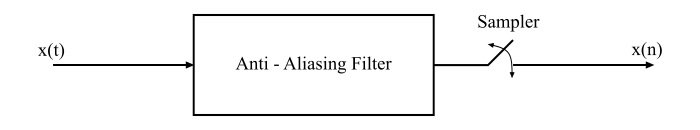# Effects of Undersampling (Aliasing) and Anti-Aliasing Filter

## What is Sampling?

The process of converting a continuous-time signal into a discrete-time signal is called sampling. Once the sampling is done, the signal is defined at discrete instants of time and the time interval between two successive sampling instants is called the sampling period.

## Nyquist Rate of Sampling

The Nyquist rate of sampling is the theoretical minimum sampling rate at which a signal can be sampled and still be reconstructed from its samples without any distortion.

## Effects of Under Sampling (Aliasing)

If a signal is sampled at less than its Nyquist rate, then it is called undersampled.

The spectrum of the sampled signal is given by,

$$\mathrm{\mathit{X_{s}\mathrm{\left(\omega \right )}\:\mathrm{=}\:\frac{\mathrm{1}}{\mathit{T}}\sum_{n\mathrm{=-\infty }}^{\infty }\mathit{X\mathrm{\left ( \mathit{\omega -n\omega _{s}} \right )}}}}$$

When a signal is undersampled, i.e.,$\mathrm{\left ( \mathit{\omega _{s}<\mathrm{2}\omega _{m}} \right )}$ where, $\mathit{\omega _{s}}$ is the sampling frequency and $\mathit{\omega _{m}}$ is the maximum frequency component present in the signal. Then, the frequency spectrum $\mathit{X\mathrm{\left ( \omega \right )}}$ of the signal $\mathit{x\mathrm{\left ( \mathit{t}\right )}}$ is no longer replicated in the spectrum of the sampled signal $\mathrm{\left[\mathit{X_{s}}\mathrm{\left (\omega \right )} \right ]}$ and hence it cannot be reconstructed by a low-pass filter. Thus, the effect in which the individual terms in the equation of $\mathit{X_{s}\mathrm{\left ( \omega \right )}}$ overlap is said to be aliasing. This process of spectral overlap is also known as frequency folding effect.

Therefore, the phenomenon in which a high-frequency component in the frequency spectrum of signal takes identity of a lower frequency component in the spectrum of the sampled signal is called aliasing.

Aliasing can occur if any of the following conditions exists −

• The sampling rate is very low.

• The signal is not band-limited to a finite range.

In order to avoid aliasing, it should be ensured that −

• $\mathit{\omega _{s}}$ is greater than $2\mathit{\omega _{m}}$.

• The signal $\mathit{x\mathrm{\left ( \mathit{t}\right )}}$ must be band-limited.

## Anti-Aliasing Filter

The low-pass filter that is used for band-limiting a signal before sampling is called the anti-aliasing filter.As the sampling theorem states that a signal can be perfectly reconstructed from its samples only when it is band-limited. But, in practice, no signal is completely band-limited, i.e., the signals have frequency spectra consisting of low-frequency components together with high-frequency noise components.

When a signal is sampled with sampling frequency ${\mathrm{\left ( \mathit{\omega _{s}} \right )}}$, then all the signals with frequency range higher than $\mathrm{\left(\mathit{\omega _{s}/\mathrm{2}} \right )}$ creating aliasing. Thus, in order to avoiding the aliasing errors caused by the undesired high-frequency signals, it is necessary to first band-limit the signal $\mathit{x\mathrm{\left ( \mathit{t} \right )}}$ to some appropriate frequency $\mathit{\omega _{m}}$ by using the low-pass filter such that most of the energy of the signal is retained. This low-pass filter (LPF) is commonly known as the anti-aliasing filter.